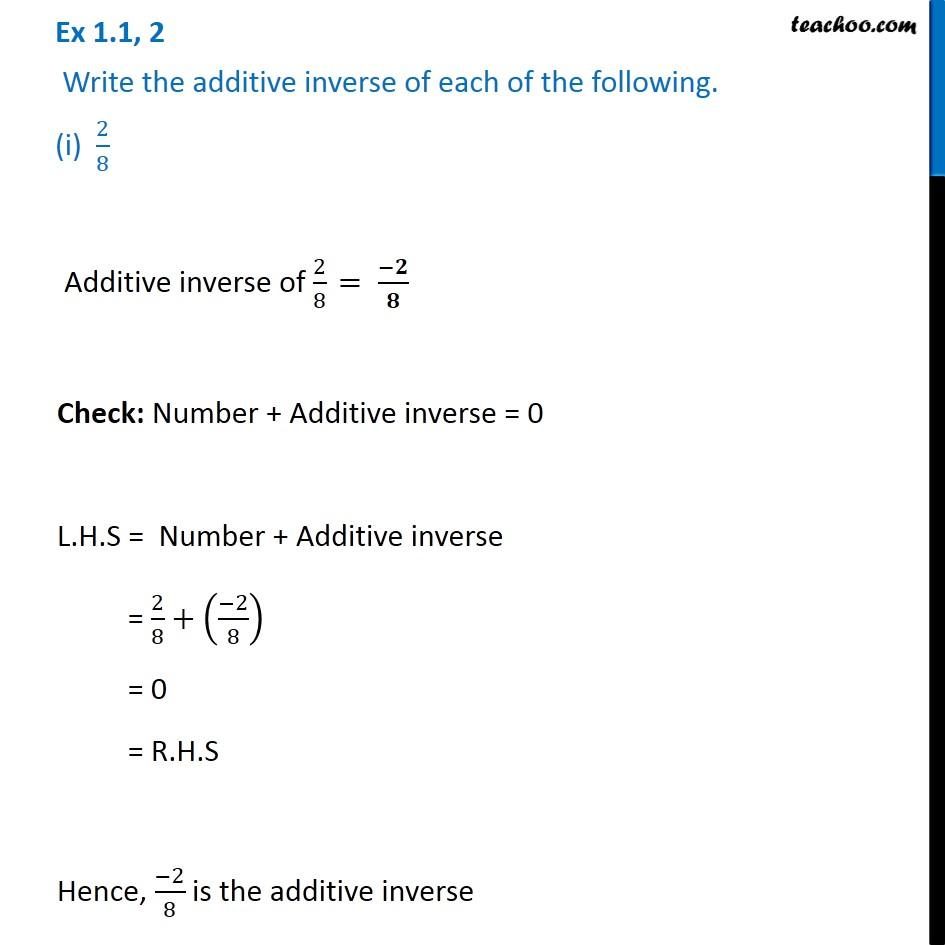Chapter 1 Class 8 Rational Numbers
Concept wiseLearn in your speed, with individual attention - Teachoo Maths 1-on-1 Class

### Transcript

Ex 1.1, 2 Write the additive inverse of each of the following. (i) 2/8 Additive inverse of 2/8 Check: Number + Additive inverse = 0 L.H.S = Number + Additive inverse = 2/8+(( 2)/8) = 0 = R.H.S Hence, ( 2)/8 is the additive inverse